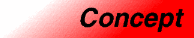# ContainerCategory: containers Component type: concept

## Description

A Container is an object that stores other objects (its elements), and that has methods for accessing its elements. In particular, every type that is a model of Container has an associated Iterators type that can be used to iterate through the Container's elements.

There is no guarantee that the elements of a Container are stored in any definite order; the order might, in fact, be different upon each iteration through the Container. Nor is there a guarantee that more than one iterator into a Container may be active at any one time. (Specific types of Containers, such as ForwardContainer, do provide such guarantees.)

A Container "owns" its elements: the lifetime of an element stored in a container cannot exceed that of the Container itself. 

## Associated types

 Value type `X::value_type` The type of the object stored in a container. The value type must be Assignable, but need not be DefaultConstructible.  Iterator type `X::iterator` The type of iterator used to iterate through a container's elements. The iterator's value type is expected to be the container's value type. A conversion from the iterator type to the const iterator type must exist. The iterator type must be an InputIterator.  Const iterator type `X::const_iterator` A type of iterator that may be used to examine, but not to modify, a container's elements.   Reference type `X::reference` A type that behaves as a reference to the container's value type.  Const reference type `X::const_reference` A type that behaves as a const reference to the container's value type.  Pointer type `X::pointer` A type that behaves as a pointer to the container's value type.  Distance type `X::difference_type` A signed integral type used to represent the distance between two of the container's iterators. This type must be the same as the iterator's distance type.  Size type `X::size_type` An unsigned integral type that can represent any nonnegative value of the container's distance type. 

## Notation

 `X` A type that is a model of Container `a`, `b` Object of type `X` `T` The value type of `X`

## Definitions

The size of a container is the number of elements it contains. The size is a nonnegative number.

The area of a container is the total number of bytes that it occupies. More specifically, it is the sum of the elements' areas plus whatever overhead is associated with the container itself. If a container's value type `T` is a simple type (as opposed to a container type), then the container's area is bounded above by a constant times the container's size times `sizeof(T)`. That is, if `a` is a container with a simple value type, then `a`'s area is `O(a.size())`.

A variable sized container is one that provides methods for inserting and/or removing elements; its size may vary during a container's lifetime. A fixed size container is one where the size is constant throughout the container's lifetime. In some fixed-size container types, the size is determined at compile time.

## Valid expressions

In addition to the expressions defined in Assignable, EqualityComparable, and LessThanComparable, the following expressions must be valid.

Name Expression Type requirements Return type
Beginning of range `a.begin()`   `iterator` if `a` is mutable, `const_iterator` otherwise  
End of range `a.end()`   `iterator` if `a` is mutable, `const_iterator` otherwise 
Size `a.size()`   `size_type`
Maximum size `a.max_size()`   `size_type`
Empty container `a.empty()`   Convertible to `bool`
Swap `a.swap(b)`   `void`

## Expression semantics

Semantics of an expression is defined only where it differs from, or is not defined in, Assignable, EqualityComparable, or LessThanComparable

Name Expression Precondition Semantics Postcondition
Copy constructor `X(a)`     `X().size() == a.size()`. `X()` contains a copy of each of `a`'s elements.
Copy constructor `X b(a);`     `b.size() == a.size()`. `b` contains a copy of each of `a`'s elements.
Assignment operator `b = a`     `b.size() == a.size()`. `b` contains a copy of each of `a`'s elements.
Destructor `a.~X()`   Each of `a`'s elements is destroyed, and memory allocated for them (if any) is deallocated.
Beginning of range `a.begin()`   Returns an iterator pointing to the first element in the container.  `a.begin()` is either dereferenceable or past-the-end. It is past-the-end if and only if `a.size() == 0`.
End of range `a.end()`   Returns an iterator pointing one past the last element in the container. `a.end()` is past-the-end.
Size `a.size()`   Returns the size of the container, that is, its number of elements.  `a.size() >= 0 && a.size() <= max_size()`
Maximum size `a.max_size()`   Returns the largest size that this container can ever have.  `a.max_size() >= 0 && a.max_size() >= a.size()`
Empty container `a.empty()`   Equivalent to `a.size() == 0`. (But possibly faster.)
Swap `a.swap(b)`   Equivalent to `swap(a,b)` 

## Complexity guarantees

The copy constructor, the assignment operator, and the destructor are linear in the container's size.

`begin()` and `end()` are amortized constant time.

`size()` is linear in the container's size.  `max_size()` and `empty()` are amortized constant time. If you are testing whether a container is empty, you should always write `c.empty()` instead of `c.size() == 0`. The two expressions are equivalent, but the former may be much faster.

`swap()` is amortized constant time. 

## Invariants

 Valid range For any container `a`, `[a.begin(), a.end())` is a valid range.  Range size `a.size()` is equal to the distance from `a.begin()` to `a.end()`. Completeness An algorithm that iterates through the range `[a.begin(), a.end())` will pass through every element of `a`.